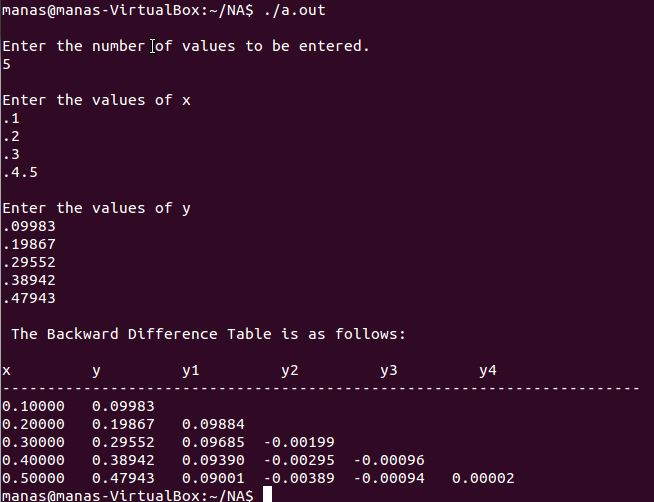# Backward Difference Table – C++ Program

```//Backward Difference
#include<iostream>
#include<iomanip>
using namespace std;
int main()
{
cout.precision(5);            //set precision
cout.setf(ios::fixed);
int i=0,j=0,n,k=0;
cout<<"\nEnter the number of values to be entered.\n";
cin>>n;
double x[n], y[n][n];            //make an array for x values and an nxn matrix for y and successive difference values, where y[n] is for the the y values
cout<<"\nEnter the values of x\n";
for (i=0;i<n;i++)
cin>>x[i];            //input x values
cout<<"\nEnter the values of y\n";
for (i=0;i<n;i++)
cin>>y[i];            //input y values in the first column of y matrix
for (j=1;j<n;j++)            //loop to calculate the difference and store them in the matrix
{
k++;
for (i=k;i<n;i++)
{
y[i][j]=y[i][j-1]-y[i-1][j-1];
}
}
cout<<"\n The Backward Difference Table is as follows: \n\n";
cout<<"x"<<setw(10)<<"y"<<setw(10);
for (i=1;i<n;i++)
cout<<"y"<<i<<setw(10);        //formatting the output and creating table headings
cout<<"\n-----------------------------------------------------------------------\n";
for (i=0;i<n;i++)            //loop for printing the diagonal matrix on the screen
{
cout<<x[i]<<setw(10);
for (j=0;j<=i;j++)
{
cout<<y[i][j];
cout<<setw(10);
}
cout<<"\n";

}
return 0;
}

```